# High School Chemistry : Solutions and Mixtures

## Example Questions

← Previous 1

### Example Question #1 : Help With Solubility Rules

Which of the following will lead to an increase in solubility of sodium chloride in water?

Decreasing the pressure of the solution

Decreasing pressure and temperature of the solution

Adding other solutes with the same chemical properties

Increasing in temperature of the solution

Lowering the temperature of the solution

Increasing in temperature of the solution

Explanation:

Solubility of solid and liquid solutes in aqueous solutions can be increased by raising the temperature of the solution. Raising the temperature increases the amount of kinetic energy of the water molecules, allowing them to better interact with the solute particles and prevent the formation of a solid lattice.

Adding other solutes with similar properties will generally decrease solubility by the common ion effect, or else will not affect solubility at all. Increasing pressure will help increase the solubility of a gas in solution, but will not affect solutions that only contain solids and liquids. Note that gas solubility will also increase with decreased temperature. This is opposite to the relationship between temperature and the solubility of solids. Low temperature will reduce the kinetic energy of gases, making them more similar to liquids, while lowering the temperature of a solid will further lower its kinetic energy and decrease its similarities with a liquid solvent.

### Example Question #1 : Solutions And Mixtures

Silver chloride is an insoluble salt when in solution. Which of the following salts will decrease the solubility of silver chloride if added to the solution?

Magnesium fluoride

Calcium bromide

Sodium bromide

Sodium chloride

Sodium chloride

Explanation:

It is possible to reduce the solubility by adding a salt that increases the concentration of an ion that is created by the insoluble salt. This is called the common ion effect.

Silver chloride will dissociate into silver and chlorine ions in solution.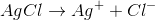If sodium chloride is added to the solution, the concentration of chlorine ions increases.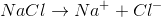This will push the equilibrium of the silver chloride dissociation to the left, thus decreasing the solubility of silver chloride.

### Example Question #3 : Help With Solubility Rules

Which of the following salts is insoluble?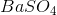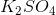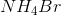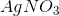Explanation:

There are some general solubility guidelines that can help you predict which salts are soluble.

1. All compounds containing ammonium, nitrate, or alkali metals are soluble.

2. Compounds with halogens as the anion are soluble. The three noted exceptions are when they are bonded to silver, mercury, or lead.

3. Sulfate compounds are soluble, except when bonded to mercury, lead, or the heavier alkaline earth metals.is an example of an insoluble sulfate compound because the sulfate is attached to barium, a heavy alkaline earth metal.

### Example Question #1 : Help With Solubility Rules

How does the temperature of a solvent affect the solubility of a solid? How does temperature of a solvent affect the solubility of a gas?

As the temperature of the solvent increases, solubility of solids decreases. As the temperature of the solvent increases, solubility of gasses decreases

As the temperature of the solvent increases, solubility of solids decreases. As the temperature of the solvent increases, solubility of gasses increases

The temperature of a solvent does not change the solubility of solids or gasses

As the temperature of the solvent increases, solubility of solids increases. As the temperature of the solvent increases, solubility of gasses increases

As the temperature of the solvent increases, solubility of solids increases. As the temperature of the solvent increases, solubility of gasses decreases

As the temperature of the solvent increases, solubility of solids increases. As the temperature of the solvent increases, solubility of gasses decreases

Explanation:

Solubility of solids increases when temperature increases because the increased kinetic energy increases the chance that a solute molecule gets surrounded by solvent molecules. Solubility for gases decreases when temperature increases because the increased kinetic energy allows for the gas molecules to break the intermolecular forces holding them in the solution, letting them bubble out of the solvent.

### Example Question #1 : Calculating Solubility

Barium fluoride is an insoluble salt.

If the solubility product constant of barium fluoride is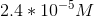, what is the solubility of barium fluoride?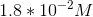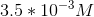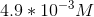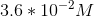Explanation:

If given the solubility product constant for a salt, we can determine the solubility for the salt as well. In order to do this, we need to use an ICE table and the equilibrium constant expression.

Initial: When the salt is added to water, there are no ions initially in solution. Because the salt is a solid, its concentration is irrelevant.

Change: When one molecule of barium fluoride dissolves, one barium ion and two fluoride ions are introduced to the solution. As a result, barium ions increase by, and fluoride ions increase by.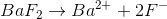End: The equilibrium expression for this salt is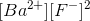, which will allow us to calculate the solubility based on the change in ion concentration.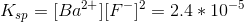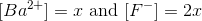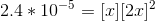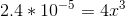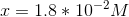The solubility for barium fluoride is.

### Example Question #1 : Solutions And Mixtures

For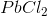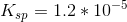Determine the maximum amount of grams ofthat will dissolve in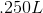of water at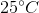.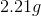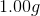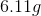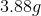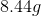Explanation:

Definition of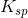: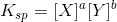for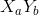.

For: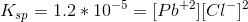Due to the chemical formula, there will be twice as many chloride ions as lead ions.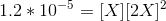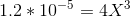Solve for the unknown variable: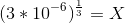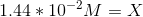Multiply times the given volume: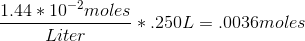Multiply times the molar mass of: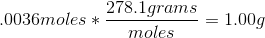### Example Question #1 : Identifying Mixtures And Solutions

What is the molarity of a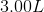solution that contains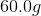of potassium nitrate?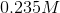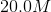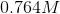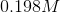Explanation:

In order to solve for molarity, we need to know the necessary equation to use. Molarity is given by the moles of solute divided by the liters of solution.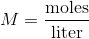Since we are given the mass of potassium nitrate, we must first convert it into moles before we can solve for the molarity of the solution. Potassium nitrate has the formula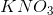. Use this to calculate the molar mass.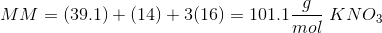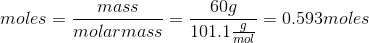Now that we have the number of moles, we can solve for the molarity of the solution using the given volume: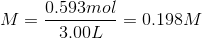### Example Question #1 : Solutions And Mixtures

Which of the following is an example of a mixture?

A glass of warm salt water

A nutrient mix for plants contains dissolved nitrogen, phosphorus, and potassium

Yeast in the bottom of a bottle of wine

A sugar cube dissolved in a cup of coffee

Yeast in the bottom of a bottle of wine

Explanation:

A mixture can be filtered or otherwise separated into its individual components. In this case, we could filter out the solid yeast from the bottom of the bottle of liquid wine. However, we could not filter out the salt, sugar, or nutrients since they are chemically mixed into the water, are in the same phase. The the salt water, coffee, and nutrient water are incorrect since these are solutions.

### Example Question #2 : Solutions And Mixtures

Which of the following is an example of a solution?

Rice cooking in a pot of water

A farmhand combining solid fertilizers in a bin

A chef making a salad out of spinach, walnuts, gorgonzola cheese, and apples

Explanation:

To identify a solution, look for a homogenous mixture composed of only one phase. Thre rice, salad, and fertilizer are descriptions of mixtures: combinations of materials which can be physically separated. The only option describing a solution is the lemonade solution since the concentrate is dissolved in water, which results in a homogenous mixture. Solutions are both mechanically and chemically combined.

### Example Question #3 : Solutions And Mixtures

Which is considered the solute when hydrochloric acid is used to disolve sodium bisulfite?

Hydrochloric acid

Sodium bisulfite

Two or more of these are correct

None of these is correct